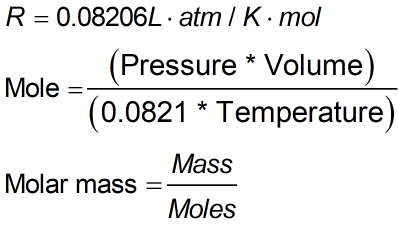Request a Tool

Molar Mass of Gas Calculator

Molar mass is a mass of a single particle of a gas multiplied by Avogadro's number

Output

Moles
0
Molar Mass of Gas
0

FormulaDefination / Uses

A substance of gas having 1 mol of mass, also narrated as grams per mole equivalent to the atom's/molecule's mass of 6.022 × 10^23, is known as the Molar Mass of Gas.

This molar mass of gas calculator uses the ideal gas law formula to calculate the molar mass of an unknown gas and the number of moles present.

If you don't have access to a molar mass of gas calculator, use caution while converting units. The constant 0.0821 is used in this equation and is meant for the following units: Temperature = Kelvin, Volume = Liters (L), and Pressure = Atmosphere (atm) (K). Use the upper given formula for manual calculations. No sign-up, registration OR captcha is required to use this tool.